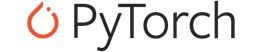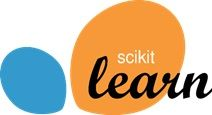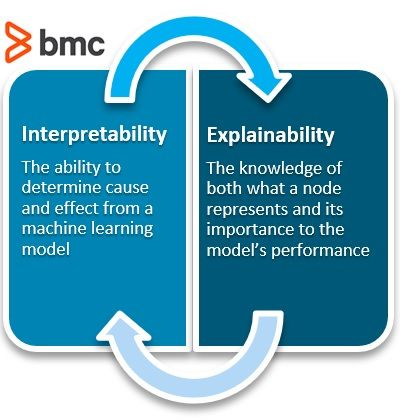﻿ Top Machine Learning Frameworks To Use in 2021 – BMC Software | Blogs
Machine Learning & Big Data Blog

# Top Machine Learning Frameworks To Use in 2021There are many machine learning frameworks. Given that each takes time to learn, and given that some have a wider user base than others, which one should you use?

In this article, we take a high-level look at the major ML frameworks ones—and some newer ones to the scene:

## What’s an ML framework?

Machine learning relies on algorithms. Unless you’re a data scientist or ML expert, these algorithms are very complicated to understand and work with.

A machine learning framework, then, simplifies machine learning algorithms. An ML framework is any tool, interface, or library that lets you develop ML models easily, without understanding the underlying algorithms.

There are a variety of machine learning frameworks, geared at different purposes. Nearly all ML the frameworks—those we discuss here and those we don’t—are written in Python. Python is the predominant machine learning programming language.

In picking a tool, you need to ask what is your goal: machine learning or deep learning? Deep learning has come to mean using neural networks to perform many tasks to analyze data:

• Image data
• Language data
• Large amounts of numbered and categorical data

Using the data, it is possible to:

• Make face-detection models
• Manipulate images, like with deep fakes
• Generate full-length, almost coherent articles on a given subject
• Predict routine behavioral actions, like when a person might cancel their gym membership
• Offer recommendations, given you like one restaurant/movie/product, here’s another you will likely enjoy

Machine learning, on the other hand, relies on algorithms based in mathematics and statistics—not neural networks—to find patterns. Most of the tutorials, use cases, and engineering in the newer ML frameworks are targeted towards building the framework that will train itself on image databases or text generation or classification in the fastest time, using the least amount of memory, and run on both GPUs and CPUs.

Why not provide just one overarching API for all ML tasks? Say, an image classification API, and let data scientists simply drop image databases into that? Or provide that as a web service, like Google’s natural language web service.

That’s because data scientists are interested in more than just handwriting recognition for the sake of handwriting recognition. Data scientists are interested in tools that solve problems applicable to business, like linear and logistic regression, k-mean clustering, and, yes, neural networks. In 2020, you have many options for these tools.

## Popular machine learning frameworks

Arguably, TensorFlow, PyTorch, and scikit-learn are the most popular ML frameworks. Still, choosing which framework to use will depend on the work you’re trying to perform. These frameworks are oriented towards mathematics and statistical modeling (machine learning) as opposed to neural network training (deep learning).

Here’s a quick breakdown of these popular ML frameworks:

• TensorFlow and PyTorch are direct competitors because of their similarity. They both provide a rich set of linear algebra tools, and they can run regression analysis.
• Scikit-learn has been around a long time and would be most familiar to R programmers, but it comes with a big caveat: it is not built to run across a cluster.
• Spark ML is built for running on a cluster, since that is what Apache Spark is all about.

Now, let’s look at some specific frameworks.

## TensorFlowTensorFlow was developed at Google Brain and then made into an open source project. TensorFlow can:

• Perform regression, classification, neural networks, etc.
• Run on both CPUs and GPUs

TensorFlow is among the de facto machine learning frameworks used today, and it is free. (Google thinks the library can be free, but ML models use significant resources for production purposes, so they capitalize on selling the resources to run their tools.)

TensorFlow is a full-blown, ML research and production tool. It can be very complex—but it doesn’t have to be. Like an Excel spreadsheet, TensorFlow can be used simply or more expertly:

• TF is simple enough for the basic user who wants to return a prediction on a given set of data
• TF can also work for the advanced user who wishes to set up multiple data pipelines, transform the data to fit their model, customize all layers and parameters of their model, and train on multiple machines while maintaining privacy of the user.

TF requires an intimate understanding of NumPy arrays. TensorFlow is built of tensors. It’s a way to process tensors; hence Python’s NumPy tool. NumPy is a Python framework for working with n-dimensional arrays (A 1-dimensional array is a vector. A 2-dimensional array is a matrix, and so forth.) Instead of doing things like automatically converting arrays to one-hot vectors (a true-false representation), this task is expected to be handled by the data scientist.

But TensorFlow has a rich set of tools. For example, the activation functions for neural networks can do all the hard work of statistics. If we define deep learning as the ability to do neural networks, then TensorFlow does that. But it can also handle more everyday problems, like regression.

A simple TF ML model looks like this:

```import tensorflow as tf
mnist = tf.keras.datasets.mnist
```
```(x_train, y_train),(x_test, y_test) = mnist.load_data()
x_train, x_test = x_train / 255.0, x_test / 255.0

model = tf.keras.models.Sequential([
tf.keras.layers.Flatten(input_shape=(28, 28)),
tf.keras.layers.Dense(128, activation='relu'),
tf.keras.layers.Dropout(0.2),
tf.keras.layers.Dense(10, activation='softmax')
])
```
```model.compile(optimizer='adam',
loss='sparse_categorical_crossentropy',
metrics=['accuracy'])

model.fit(x_train, y_train, epochs=5)
model.evaluate(x_test, y_test)```

A simple model and more advanced model can be seen here.

## PyTorchPyTorch was developed by FAIR, Facebook AI Research. In early 2018, the FAIR team merged Caffe2, another ML framework, into PyTorch. It is the leading competitor to TensorFlow. When engineers are deciding to use a ML platform, their choice generally comes down to, “Do we use TensorFlow or PyTorch?” They each serve their purposes but are pretty interchangeable.

Like TensorFlow, PyTorch:

• Does regression, classification, neural networks, etc.
• Runs on both CPUs and GPUs.

PyTorch is considered more pythonic. Where TensorFlow can get a model up and running faster and with some customization, PyTorch is considered more customizable, following a more traditional object-oriented programming approach through building classes.

PyTorch is shown to have faster training times. This speed is marginal for many users but can make a difference on large projects. PyTorch and TensorFlow are both in active development, so the speed comparison is likely to waiver back and forth between the two.

Relative to Torch, PyTorch uses Python and has no need for Lua or the Lua Package Manager.
From Asad Mahmood, a PyTorch model looks like this:

```import torch
def __init__(self, inputSize, outputSize):
super(linearRegression, self).__init__()
self.linear = torch.nn.Linear(inputSize, outputSize)

def forward(self, x):
out = self.linear(x)
return out
```
```inputDim = 1 # takes variable 'x'
outputDim = 1 # takes variable 'y'
learningRate = 0.01
epochs = 100

model = linearRegression(inputDim, outputDim)
##### For GPU #######
if torch.cuda.is_available():
model.cuda()
```
```criterion = torch.nn.MSELoss()
optimizer = torch.optim.SGD(model.parameters(), lr=learningRate)
```
```for epoch in range(epochs):
# Converting inputs and labels to Variable
if torch.cuda.is_available():
inputs = Variable(torch.from_numpy(x_train).cuda())
labels = Variable(torch.from_numpy(y_train).cuda())
else:
inputs = Variable(torch.from_numpy(x_train))
labels = Variable(torch.from_numpy(y_train))

# Clear gradient buffers because we don't want any gradient from previous epoch to carry forward, dont want to cummulate gradients

```

```   # get output from the model, given the inputs
outputs = model(inputs)

# get loss for the predicted output
loss = criterion(outputs, labels)
print(loss)
# get gradients w.r.t to parameters
loss.backward()

# update parameters
optimizer.step()

print('epoch {}, loss {}'.format(epoch, loss.item()))
```

## scikit-LearnSometimes, only a quick test is needed to measure the likely success of a hypothesis. Scikit-learn is an old standards of the data science world, and it can be good to run quick ML model sketches, to see if a model might have some interpretability.Scikit is another Python package that can perform many useful machine learning tasks:

• Linear regression
• Decision tree regressions
• Random Forest regressions
• K-Nearest neighbor
• SVMs
• And more

Scikit provides model analysis tools like the confusion matrix for assessing how well a model performed. Many times, you can start an ML job in scikit-learn and then move to another framework. For example, scikit-learn has excellent data pre-processing tools for one-hot encoding categorical data. Once the data is pre-processed through Scikit, you can move it into TensorFlow or PyTorch.

```from sklearn import linear_model

regr = linear_model.LinearRegression()
regr.fit(diabetes_X_train, diabetes_y_train)
print(regr.coef_)
```

## Spark ML

We have written at length about how to use Spark ML. As we described earlier, Spark ML can run in clusters. In other words, it can handle really large matrix multiplication by taking slices of the matrix and running that calculation on different servers. (Matrix multiplication is among the most important ML operations.) That requires a distributed architecture, so your computer does not run out of memory or run too long when working with large amounts of data.

Spark ML is complicated, but instead of having to work with NumPy arrays, it lets you work with Spark RDD data structures, which anyone using Spark in its big data role will understand. And you can use Spark ML to work with Spark SQL dataframes, which most Python programmers know. So it creates dense and spark feature-label vectors for you, taking away some complexity of preparing data to feed into the ML algorithms.

In January 2019, Yahoo released TensorFlowOnSpark, a library that “combines salient features from the TensorFlow deep Learning framework with Apache Spark and Apache Hadoop. TensorFlowOnSpark enables distributed deep learning on a cluster of GPU and CPU servers.” The package integrates big data and machine learning into a good-to-use ML tool for large production use-cases.

The Spark ML model, written in Scala or Java, looks similar to the TensorFlow code, in that it is more declarative:

```// Build the model with the desired layers
val training = sparkContext.parallelize(Seq(
LabeledPoint(1.0, Vectors.dense(0.0, 1.1, 0.1)),
LabeledPoint(0.0, Vectors.dense(2.0, 1.0, -1.0)),
LabeledPoint(0.0, Vectors.dense(2.0, 1.3, 1.0)),
LabeledPoint(1.0, Vectors.dense(0.0, 1.2, -0.5))))
val lr = new LogisticRegression()
```
```// We may set parameters using setter methods.
lr.setMaxIter(10)
.setRegParam(0.01)
```

```// Train a LogisticRegression model.  This uses the parameters stored in lr.
val model1 = lr.fit(training)
```

## TorchTorch claims to be the easiest ML framework. It is an old machine learning library, first released in 2002.

Before, with PyTorch, Python was the chosen method to access the fundamental tables in which Torch performs its calculations. Torch itself can be used using Lua, with the LuaRocks Package Manager. Torch’s relative simplicity comes from its Lua programming language interface (There are other interfaces, like QT, and iPython/Jupyter, and it has a C implementation.). Lua is indeed simple. There are no floats or integers, just numbers. And all objects in Lua are tables. So, it’s easy to create data structures. And it provides a rich set of easy-to-understand features to slice tables and add to them.

Like TensorFlow, the basic data element in Torch is the tensor. You create one by writing torch.Tensor. The CLI (command line interface) provides inline help and it helps with indentation. People who have used Python will be relieved, as this means you can type functions in situ without having to start over at the beginning when you make a mistake. And for those who like complexity and sparse code, Torch supports functional programming.

## New ML framework types

The Machine Learning world is rich with libraries. There exist high-level libraries which use some of these previous mentioned libraries as their base in order to make machine learning easier for the data scientist.

### huggingface.co

One of the top machine learning libraries is huggingface.co’s, which creates good base models for researchers built on top of TensorFlow and PyTorch. They adapt complicated tools, such as GPT-2, to work easily on your machine.

### Keras

Keras is a neural network library built on top of TensorFlow to make ML modelling straightforward. It simplifies some of the coding steps, like offering all-in-one models, Keras can also use the same code to run on a CPU or a GPU.

Keras isn’t limited to TensorFlow, though it’s most commonly used there. You can also use Keras with:

• Microsoft Cognitive Toolkit (CNTK)
• R
• Theano
• PlaidML

For more on machine learning, explore the BMC Machine Learning & Big Data Blog and these resources:

This e-book teaches machine learning in the simplest way possible. This book is for managers, programmers, directors – and anyone else who wants to learn machine learning. We start with very basic stats and algebra and build upon that.

These postings are my own and do not necessarily represent BMC's position, strategies, or opinion.

See an error or have a suggestion? Please let us know by emailing blogs@bmc.com.

### BMC Bring the A-Game

From core to cloud to edge, BMC delivers the software and services that enable nearly 10,000 global customers, including 84% of the Forbes Global 100, to thrive in their ongoing evolution to an Autonomous Digital Enterprise.#### Walker Rowe

Walker Rowe is an American freelancer tech writer and programmer living in Cyprus. He writes tutorials on analytics and big data and specializes in documenting SDKs and APIs. He is the founder of the Hypatia Academy Cyprus, an online school to teach secondary school children programming. You can find Walker here and here.